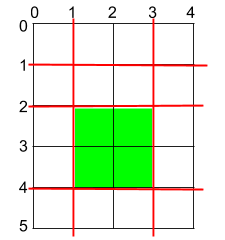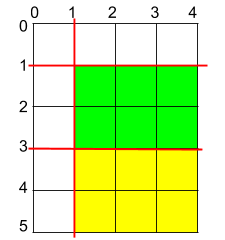##### Welcome to Subscribe On Youtube

Formatted question description: https://leetcode.ca/all/1465.html

# 1465. Maximum Area of a Piece of Cake After Horizontal and Vertical Cuts

Medium

## Description

Given a rectangular cake with height h and width w, and two arrays of integers horizontalCuts and verticalCuts where horizontalCuts[i] is the distance from the top of the rectangular cake to the ith horizontal cut and similarly, verticalCuts[j] is the distance from the left of the rectangular cake to the jth vertical cut.

Return the maximum area of a piece of cake after you cut at each horizontal and vertical position provided in the arrays horizontalCuts and verticalCuts. Since the answer can be a huge number, return this modulo 10^9 + 7.

Example 1:Input: h = 5, w = 4, horizontalCuts = [1,2,4], verticalCuts = [1,3]

Output: 4

Explanation: The figure above represents the given rectangular cake. Red lines are the horizontal and vertical cuts. After you cut the cake, the green piece of cake has the maximum area.

Example 2:Input: h = 5, w = 4, horizontalCuts = [3,1], verticalCuts = 

Output: 6

Explanation: The figure above represents the given rectangular cake. Red lines are the horizontal and vertical cuts. After you cut the cake, the green and yellow pieces of cake have the maximum area.

Example 3:

Input: h = 5, w = 4, horizontalCuts = , verticalCuts = 

Output: 9

Constraints:

• 2 <= h, w <= 10^9
• 1 <= horizontalCuts.length < min(h, 10^5)
• 1 <= verticalCuts.length < min(w, 10^5)
• 1 <= horizontalCuts[i] < h
• 1 <= verticalCuts[i] < w
• It is guaranteed that all elements in horizontalCuts are distinct.
• It is guaranteed that all elements in verticalCuts are distinct.

## Solution

Sort both arrays horizontalCuts and verticalCuts. For the first and the last element of each array, calculate the absolute difference with 0 or with h and w, respectively. Then each time calculate the absolute difference between each pair of adjacent elements in horizontalCuts and verticalCuts respectively. Find the maximum absolute difference in horizontal direction and in vertical direction respectively, and calculate the product.

• class Solution {
public int maxArea(int h, int w, int[] horizontalCuts, int[] verticalCuts) {
final int MODULO = 1000000007;
Arrays.sort(horizontalCuts);
Arrays.sort(verticalCuts);
int horizontalLength = horizontalCuts.length, verticalLength = verticalCuts.length;
int maxHorizontal = Math.max(horizontalCuts, h - horizontalCuts[horizontalLength - 1]);
int maxVertical = Math.max(verticalCuts, w - verticalCuts[verticalLength - 1]);
for (int i = 1; i < horizontalLength; i++) {
int horizontal = horizontalCuts[i] - horizontalCuts[i - 1];
maxHorizontal = Math.max(maxHorizontal, horizontal);
}
for (int i = 1; i < verticalLength; i++) {
int vertical = verticalCuts[i] - verticalCuts[i - 1];
maxVertical = Math.max(maxVertical, vertical);
}
long maxArea = (long) maxHorizontal * (long) maxVertical;
return (int) (maxArea % MODULO);
}
}

• class Solution:
def maxArea(
self, h: int, w: int, horizontalCuts: List[int], verticalCuts: List[int]
) -> int:
horizontalCuts.extend([0, h])
verticalCuts.extend([0, w])
horizontalCuts.sort()
verticalCuts.sort()
x = max(b - a for a, b in pairwise(horizontalCuts))
y = max(b - a for a, b in pairwise(verticalCuts))
return (x * y) % (10**9 + 7)

############

class Solution:
def maxArea(self, h: int, w: int, horizontalCuts: List[int], verticalCuts: List[int]) -> int:
horizontalCuts.append(0); horizontalCuts.append(h)
verticalCuts.append(0); verticalCuts.append(w)
horizontalCuts.sort()
verticalCuts.sort()
M, N = len(horizontalCuts), len(verticalCuts)
max_hc = 0
max_vc = 0
for i in range(M - 1):
max_hc = max(max_hc, horizontalCuts[i + 1] - horizontalCuts[i])
for j in range(N - 1):
max_vc = max(max_vc, verticalCuts[j + 1] - verticalCuts[j])
return (max_hc * max_vc) % (10 ** 9 + 7)

• class Solution {
public:
int maxArea(int h, int w, vector<int>& horizontalCuts, vector<int>& verticalCuts) {
horizontalCuts.push_back(0);
horizontalCuts.push_back(h);
verticalCuts.push_back(0);
verticalCuts.push_back(w);
sort(horizontalCuts.begin(), horizontalCuts.end());
sort(verticalCuts.begin(), verticalCuts.end());
int x = 0, y = 0;
for (int i = 1; i < horizontalCuts.size(); ++i) {
x = max(x, horizontalCuts[i] - horizontalCuts[i - 1]);
}
for (int i = 1; i < verticalCuts.size(); ++i) {
y = max(y, verticalCuts[i] - verticalCuts[i - 1]);
}
int mod = 1e9 + 7;
return (int) ((1ll * x * y) % mod);
}
};

• func maxArea(h int, w int, horizontalCuts []int, verticalCuts []int) int {
horizontalCuts = append(horizontalCuts, []int{0, h}...)
verticalCuts = append(verticalCuts, []int{0, w}...)
sort.Ints(horizontalCuts)
sort.Ints(verticalCuts)
x, y := 0, 0
mod := int(1e9) + 7
for i := 1; i < len(horizontalCuts); i++ {
x = max(x, horizontalCuts[i]-horizontalCuts[i-1])
}
for i := 1; i < len(verticalCuts); i++ {
y = max(y, verticalCuts[i]-verticalCuts[i-1])
}
return (x * y) % mod
}

func max(a, b int) int {
if a > b {
return a
}
return b
}